## Monday, May 20, 2019

### Lots of updates to the new learnPopGen package, just submitted to CRAN

<code>clt</code>: <i>Illustrates the concept of the Central Limit Theorem</i>

I just updated my R package learnPopGen and submitted to CRAN.

While waiting for the new release to percolate through all the mirror CRAN repository, users can update their local version from the learnPopGen GitHub page using devtools as follows:

``````library(devtools)
install_github("liamrevell/learnPopGen")
``````
``````library(learnPopGen)
packageVersion("learnPopGen")
``````
``````##  '1.0.4'
``````

There are a ton of new features in this new version. Most notably, the majority of the functions of the package now invisibly return their data (that is, the results from numerical analysis or simulation) to the user in the form of a special object class, that can be printed, inspected, re-plotted, or combined with other graphs. This is neat because it means that we can, for instance, visualize the result of a set of genetic drift simulations conducted using the function `genetic.drift`, but save these data to an object, and then re-plot them in combination with a graph showing the mean heterozygosity through time compared to the theoretical expectations (shown below). We could have done this before but we would have had to set the seed and then conducted our genetic drift simulation twice.

Below I'll review some of the different functions of the package. This leaves out functions or function methods that necessarily involve animation - because that becomes somewhat complicated to embed on the blog.

## `clt`: Illustrates the concept of the Central Limit Theorem

``````object<-clt(nvar=10,df="exponential")
``````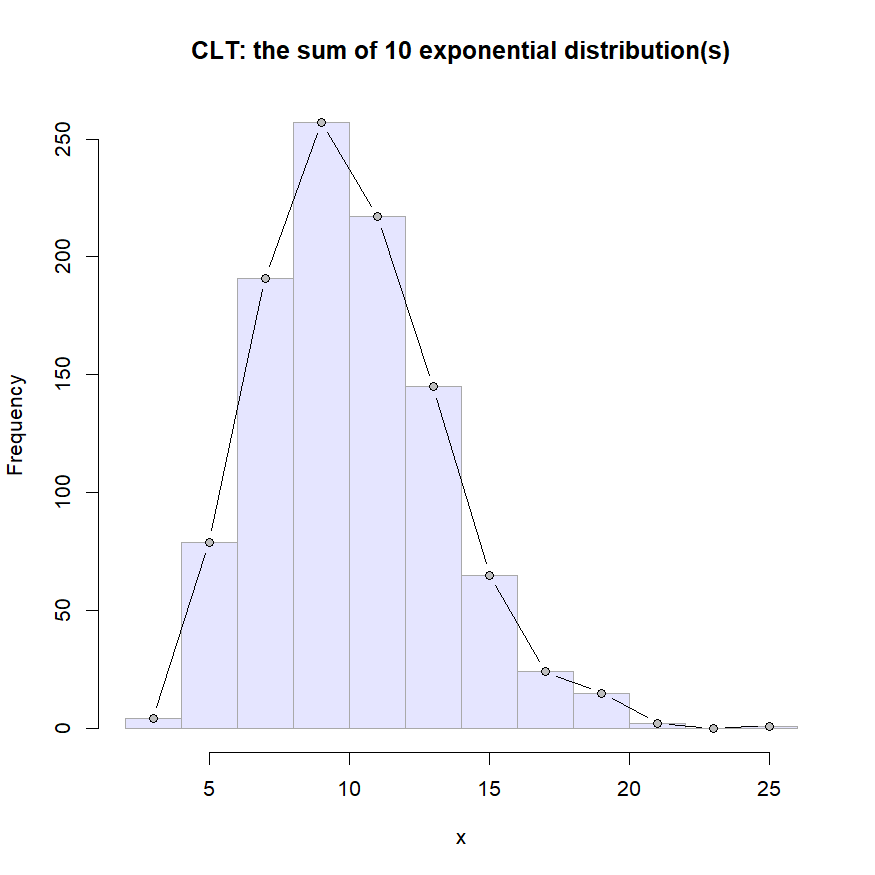``````print(object)
``````
``````##
## Object of class "clt" consisting of:
##   (1) 10 exponentially distributed independent random variables, each with
##   (2) 1000 observations (stored in x\$data), and
##   (3) a histogram giving the distribution of their observation-wise sum.
``````
``````par(mfrow=c(3,3),cex.main=0.8)
df<-c("uniform", "exponential", "binomial")
nvar<-c(1, 4, 20)
for(i in 1:length(nvar))
for(j in 1:length(df))
clt(nvar=nvar[i],nobs=10000,df=df[j])
``````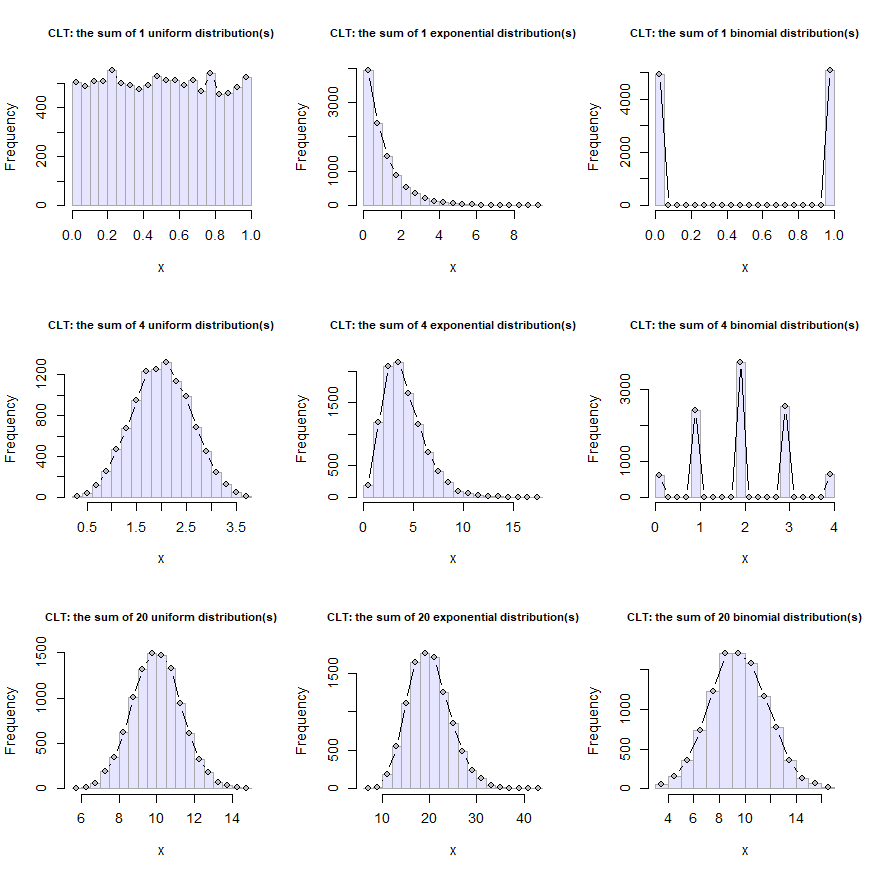## `coalescent.plot`: Creates a (usually animated) simulation of gene coalescence within a population

``````object<-coalescent.plot(n=20,ngen=20,col.order="alternating")
``````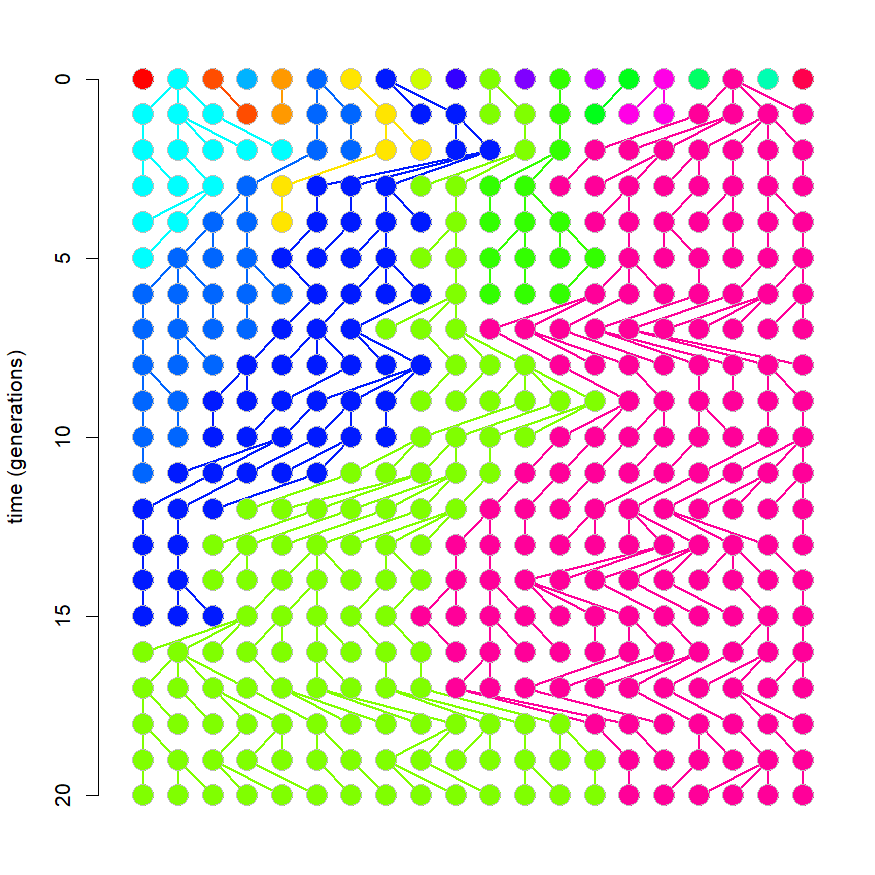``````object
``````
``````##
## Object of class "coalescent.plot" consisting of a simulated process of allelic
## coalescence over 20 generations, in a population containing 20 individuals.
##
## The object consists of:
##   (1) a 21 x 20 numeric matrix containing the unique 'alleles'
##       present in the population from time=0 to time=20.
##   (2) a 20 x 20 numeric matrix giving the parent/offspring
##       relationships across all 20 generations of the simulation.
##
## To re-plot type plot(object_name) at the command line.
``````
``````plot(object,col.order="sequential")
``````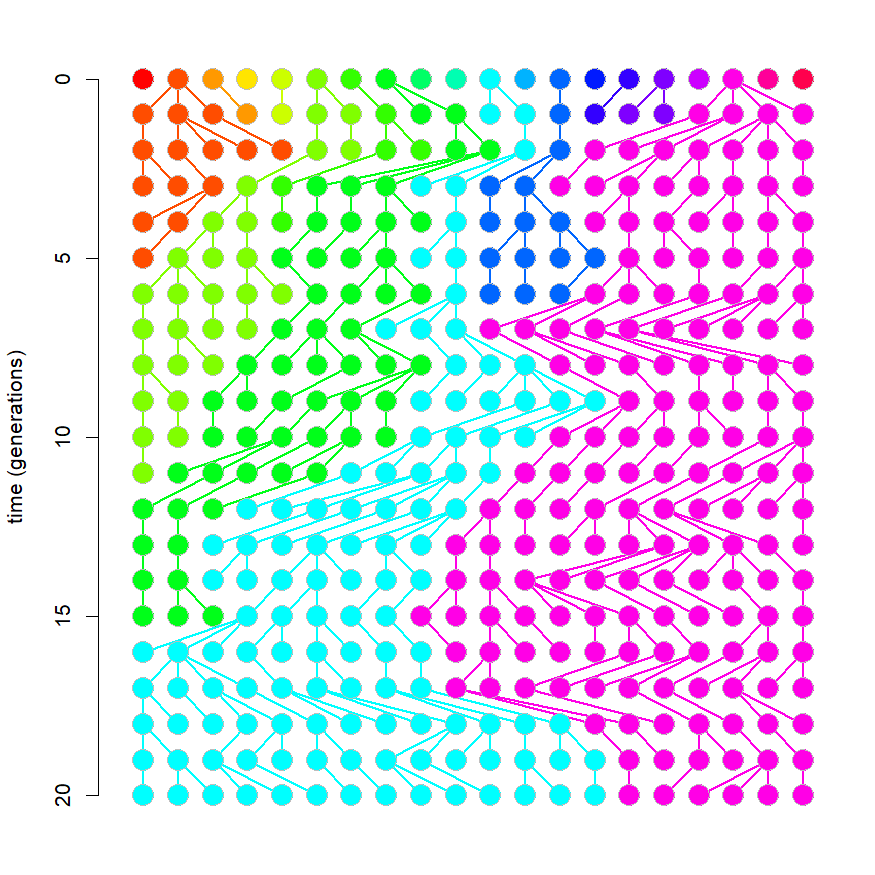## `drift.selection`: Simulation of genetic drift & natural selection at a biallelic locus

``````object<-drift.selection(p0=0.4,w=c(1,0.8,0.95),ngen=50)
``````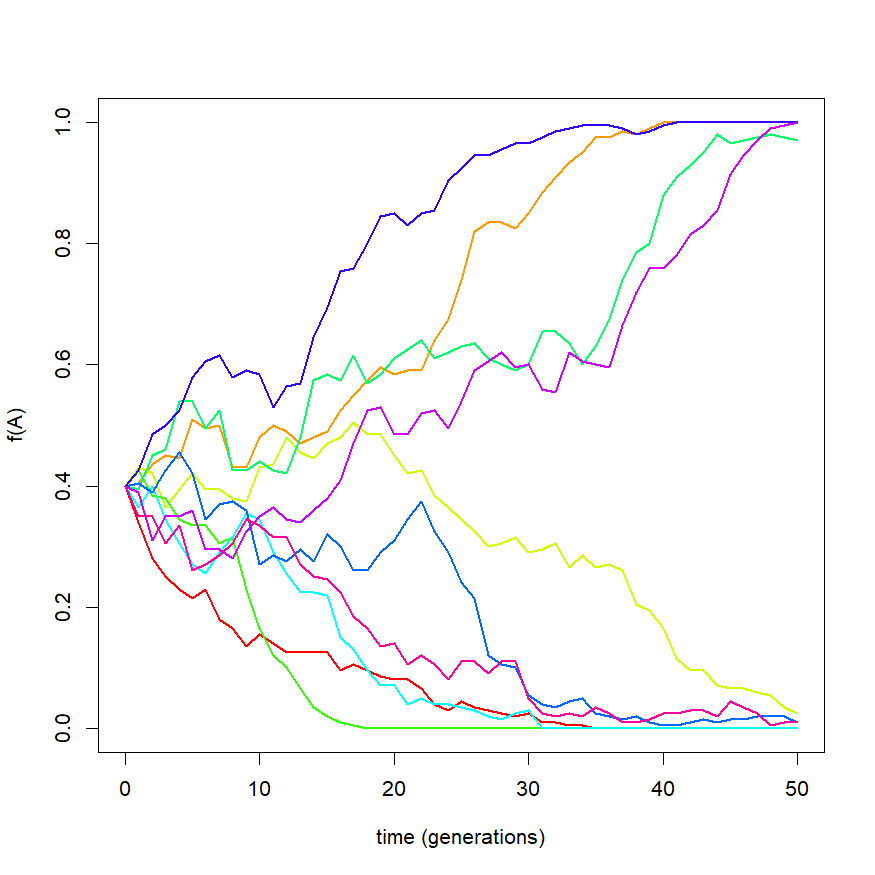``````object
``````
``````##
## Object of class "drift.selection" consisting of
## allele frequencies through time from 10 simulation(s) of
## 50 generations of genetic drift & natural selection
## with normalized fitnesses of:
##     W(AA) = 1
##     W(Aa) = 0.8
##     W(aa) = 0.95
##
## To plot enter plot('object_name') at the command line
## interface.
``````
``````plot(object,type="s",colors=grey(seq(0,0.9,by=0.1)))
``````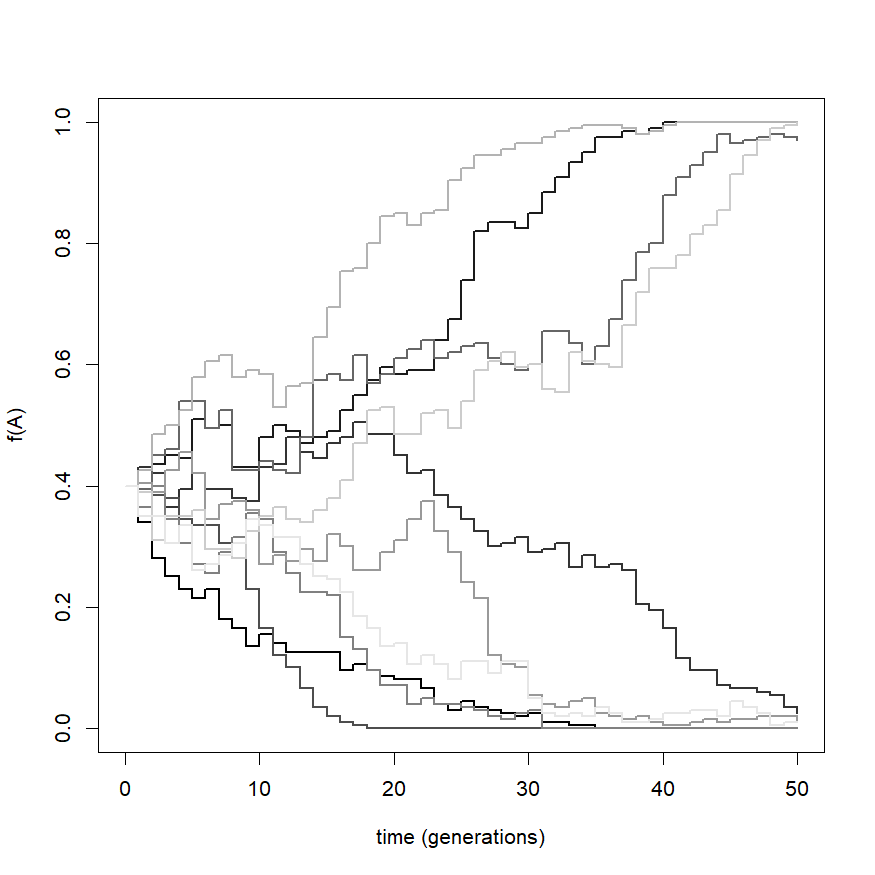## `founder.event`: Simulation of a founder event or population bottleneck

``````object<-founder.event(p0=0.5,Nf=2,ttime=100,etime=c(45,55))
``````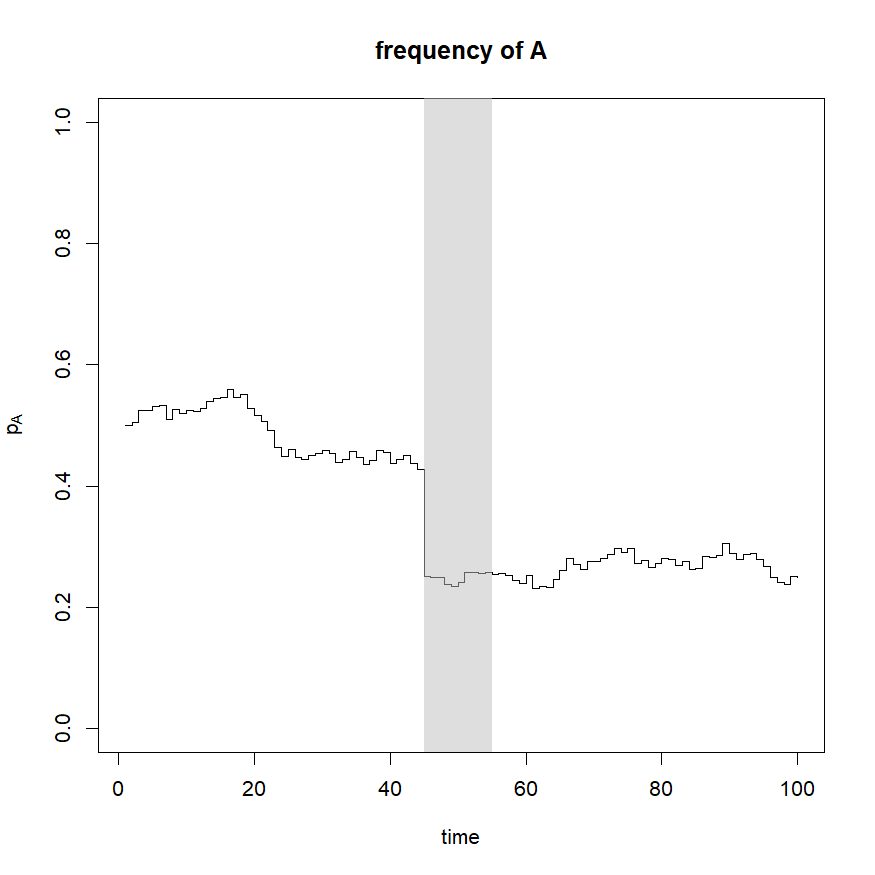``````object
``````
``````##
## Object of class "founder.event" consisting of 100
## total generations, including a bottleneck of 10
## generations.
## The simulation had an effective population size of 1000
## and a founder population size of 2.
##
## To plot enter plot('object_name') at the command line
## interface.
``````
``````par(mfrow=c(2,1),mar=c(4.1,5.1,2.1,1.1))
plot(object,show="p")
plot(object,show="var")
``````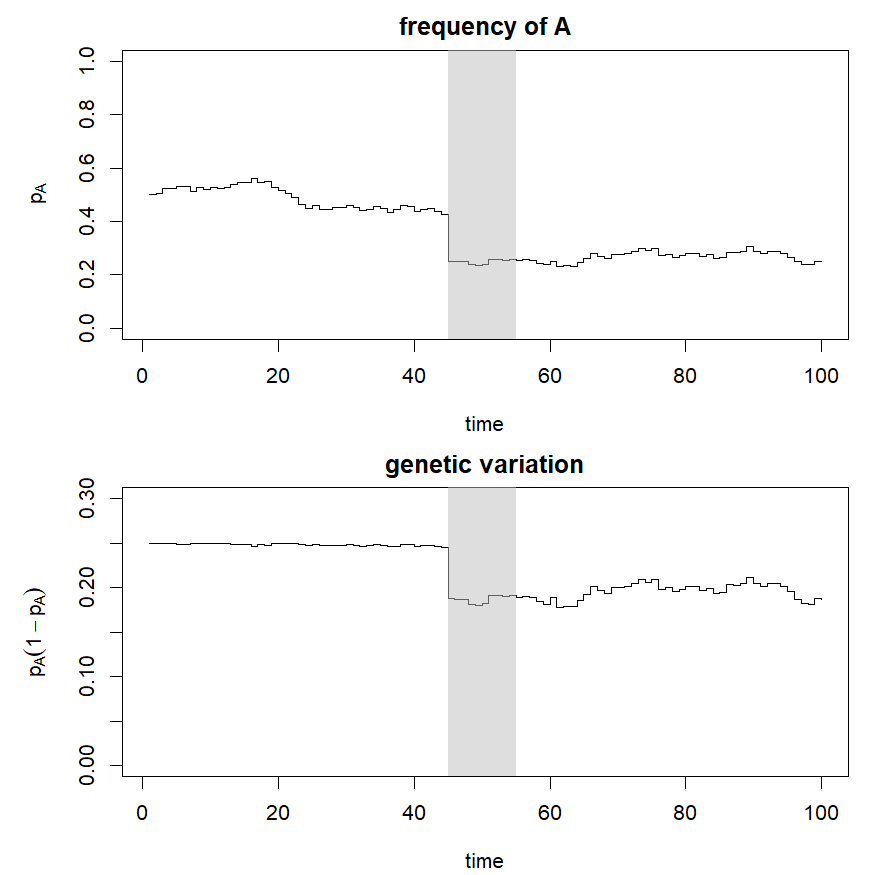## `freqdep`: Numerical analysis of a frequency dependent selection model

``````object<-freqdep(s=2.1)
``````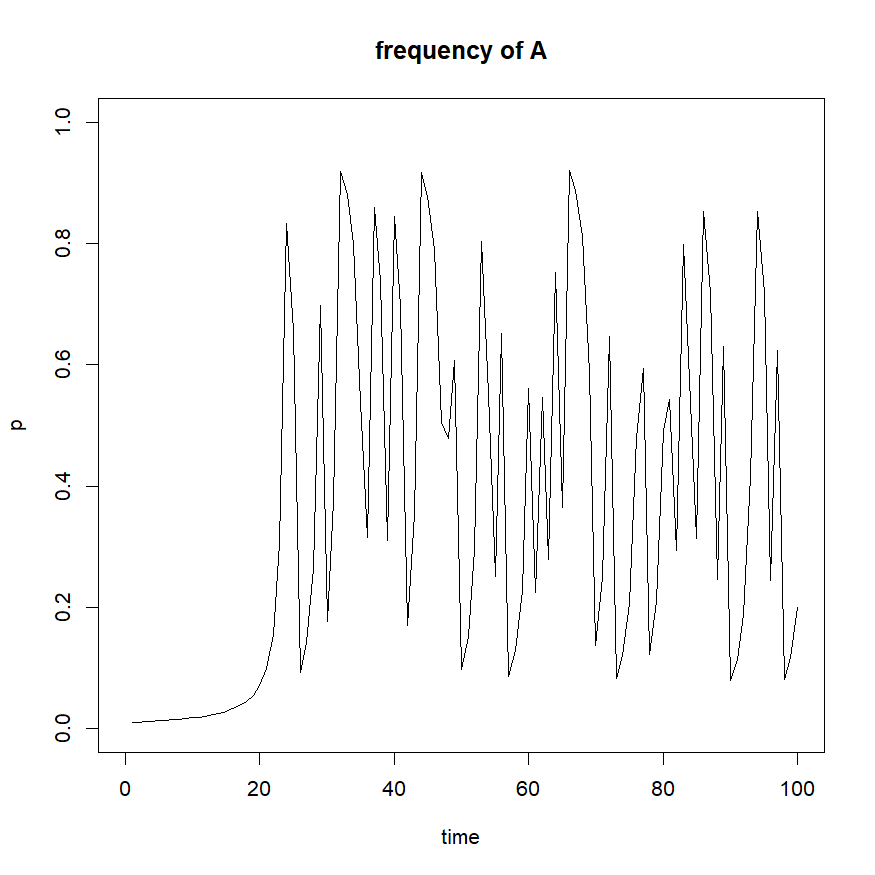``````par(mfrow=c(2,2))
plot(object,show="p")
freqdep(s=2.1,show="fitness")
freqdep(s=2.1,show="deltap")
freqdep(s=2.1,show="cobweb")
``````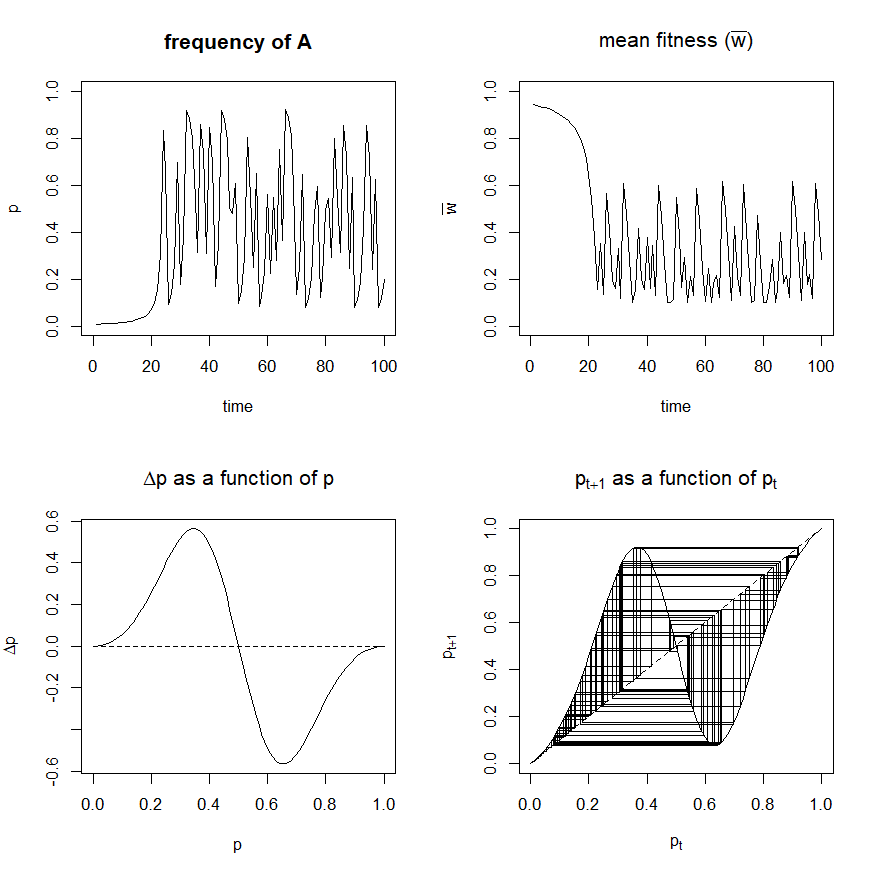## `genetic.drift`: Genetic drift simulation

``````object<-genetic.drift(Ne=100,time=200,nrep=20,pause=0)
``````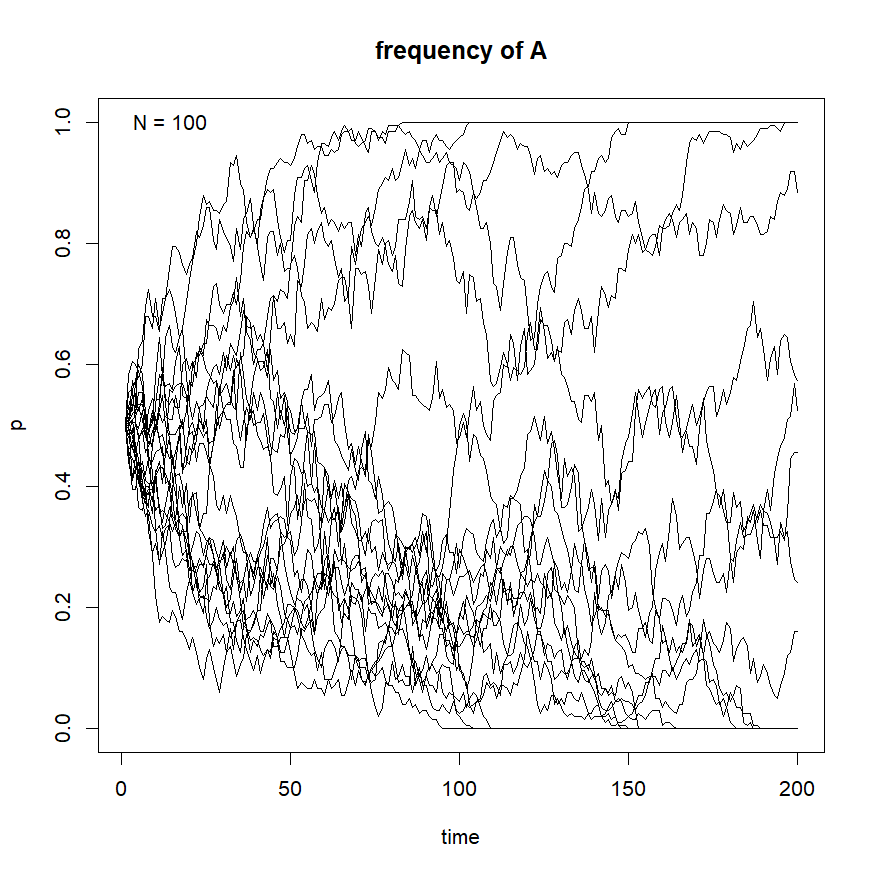``````object
``````
``````##
## Object of class "genetic.drift" consisting of allele frequencies
## from 20 independent genetic drift simulation(s) each initiated
## with a starting allele frequency of 0.5 and an effective population
## size of 100.
##
##
## To plot enter plot('object_name') at the command line interface.
``````
``````par(mfrow=c(2,1),mar=c(4.1,4.1,2.1,1.1))
plot(object,show="p",type="s")
plot(object,show="heterozygosity",col="blue")
``````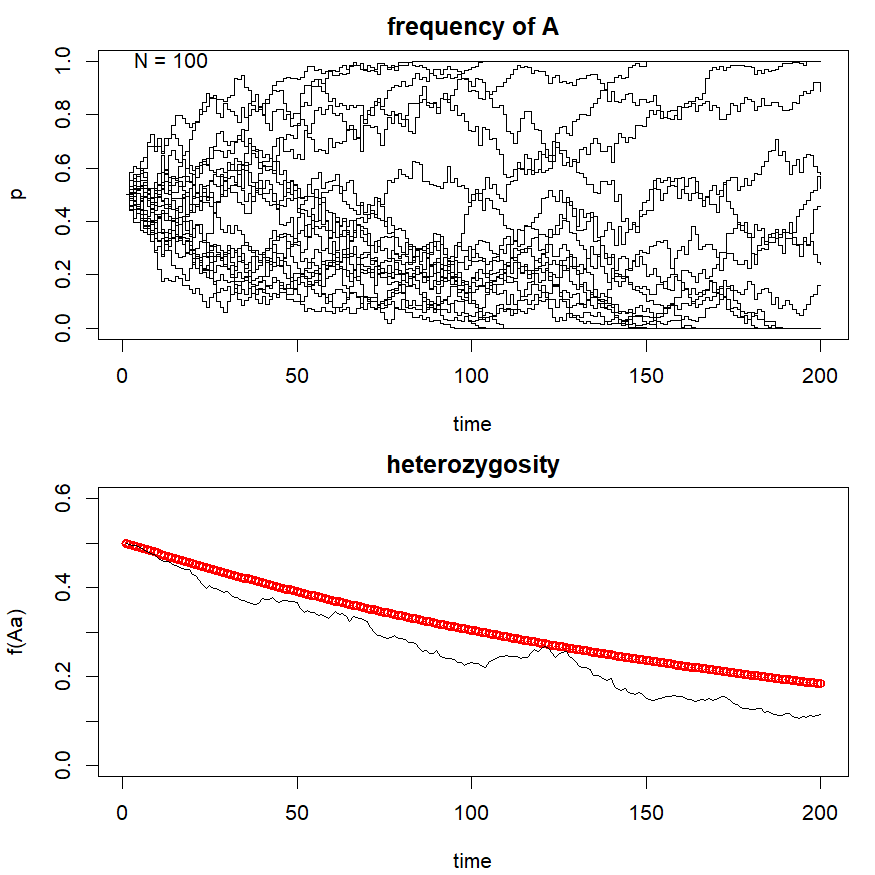## `hawk.dove`: Analysis of hawk-dove game theoretic model

``````Payoff<-matrix(c(0.5,0.6,1.5,1.0),2,2)
object<-hawk.dove(M=Payoff,time=60)
``````
``````## Pay-off matrix:
``````
``````##      hawk dove
## hawk  0.5  1.5
## dove  0.6  1.0
``````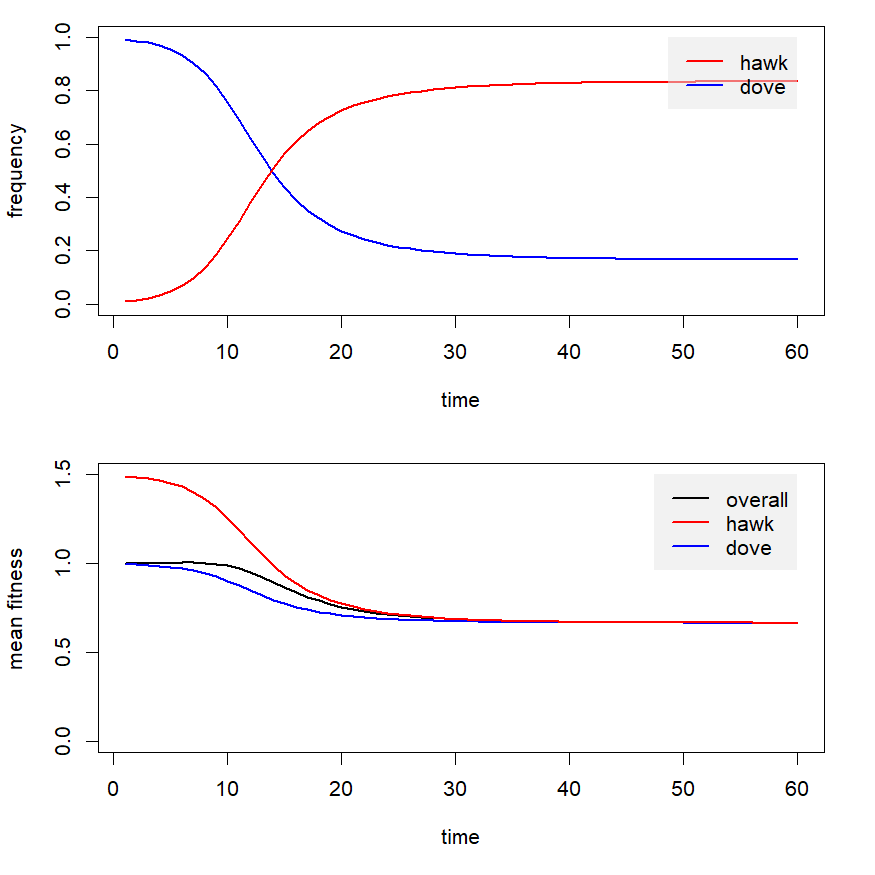``````object
``````
``````##
## Object of class "hawk.dove" consisting of the results of a
## numerical analysis of the so-called Hawk-Dove model in which
## fitness is assumed to be proportional to the payoff from
## interactions between the two alternative strategies as given
## by the matrix:
##      hawk dove
## hawk  0.5  1.5
## dove  0.6  1.0
##
##
## To plot enter plot('object_name') at the command line
## interface.
``````

## `msd`: Migration, drift, and selection

``````par(mfrow=c(3,1))
msd(p0=c(0.5,0.5),w=list(c(1,0.9,0.8),
c(0.8,0.9,1)),m=c(0.01,0.01),ngen=100)
msd(p0=c(0.5,0.5),w=list(c(1,0.9,0.8),
c(0.8,0.9,1)),m=c(0.05,0.05),ngen=100)
msd(p0=c(0.5,0.5),w=list(c(1,0.9,0.8),
c(0.8,0.9,1)),m=c(0.1,0.1),ngen=100)
``````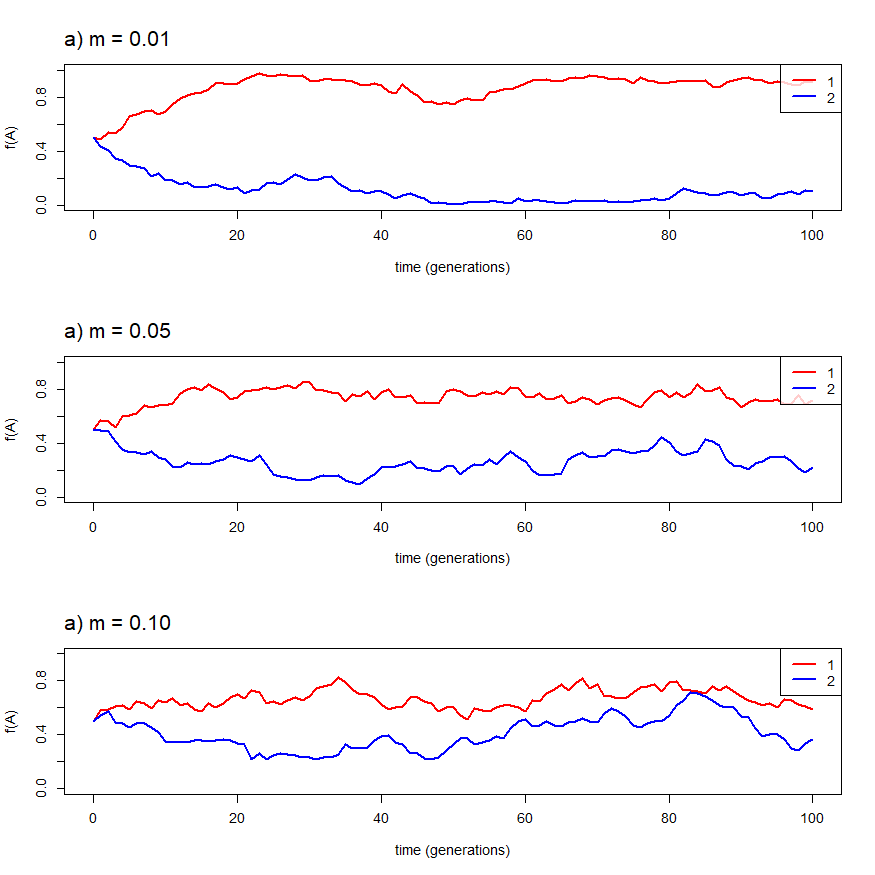## `phenotype.freq`: Computes phenotypic distribution for a polygenic trait

``````object<-phenotype.freq(nloci=6,p=rep(0.5,6),effect=1/6)
``````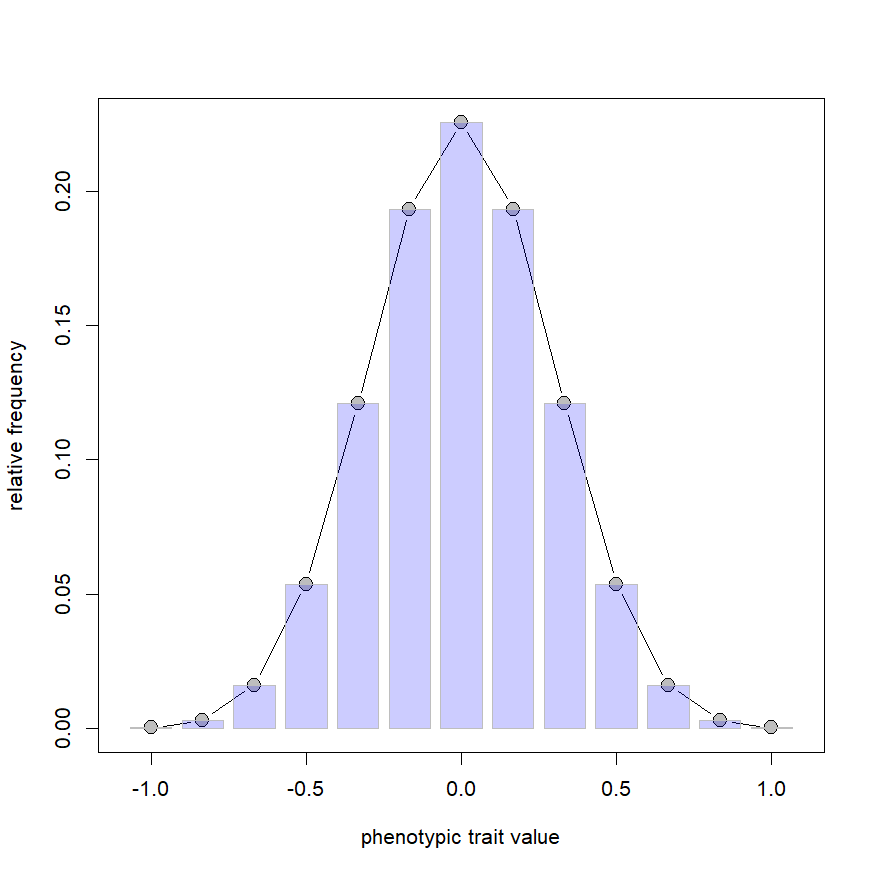``````object
``````
``````##
## Object of class "phenotype.freq" containing the frequencies of each value
## of a hypothetical polygenic trait determined by the additive effect of 6
## genetic loci with the following frequencies,
##   p(A): 0.5, 0.5, 0.5, 0.5, 0.5, 0.5
## and the following additive effect of allelic subsitution,
##   a: 0.17, 0.17, 0.17, 0.17, 0.17, 0.17
##
##
## To plot enter plot('object_name') at the command line interface.
``````
``````object<-phenotype.freq(nloci=10,p=runif(n=10),effect=rexp(n=10))
``````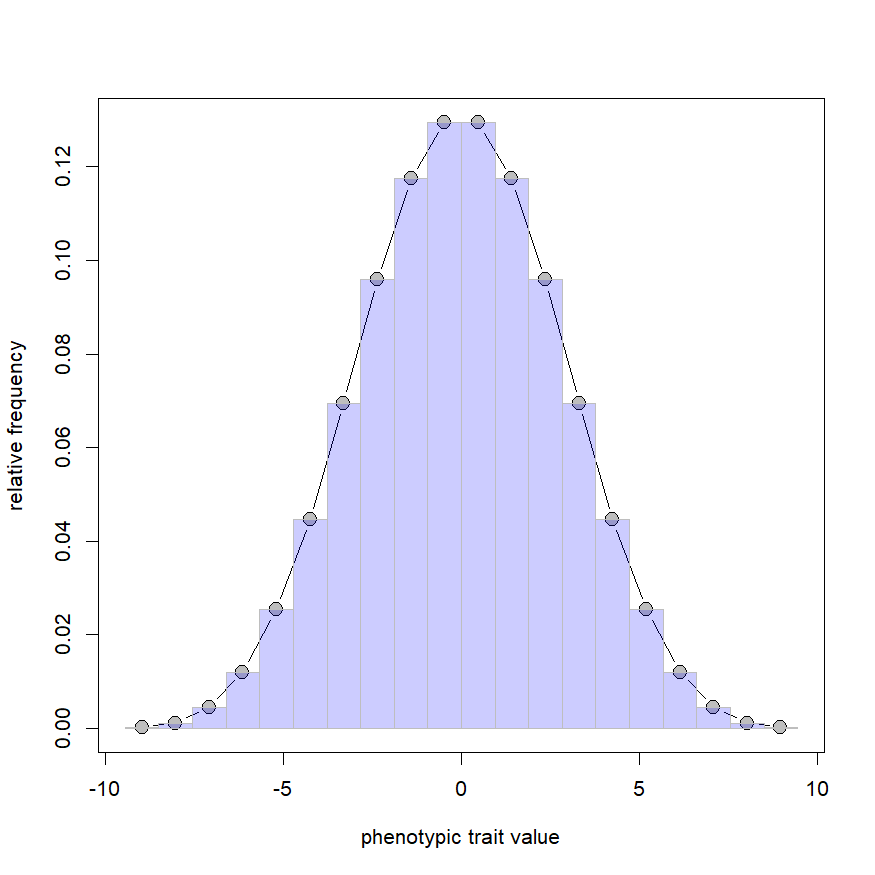``````object
``````
``````##
## Object of class "phenotype.freq" containing the frequencies of each value
## of a hypothetical polygenic trait determined by the additive effect of 10
## genetic loci with the following frequencies,
##   p(A): 0.19, 0.65, 0.27, 0.75, 0.85, 0.64, 0.33, 0.4, 0.43, 0.68
## and the following additive effect of allelic subsitution,
##   a: 0.85, 0.64, 0.51, 1.71, 1.64, 0.66, 0.75, 0.66, 0.37, 1.66
##
##
## To plot enter plot('object_name') at the command line interface.
``````

## `selection`: Numerical analysis of biallelic locus frequency independent selection

``````object<-selection(w=c(0.8,1.1,0.7))
``````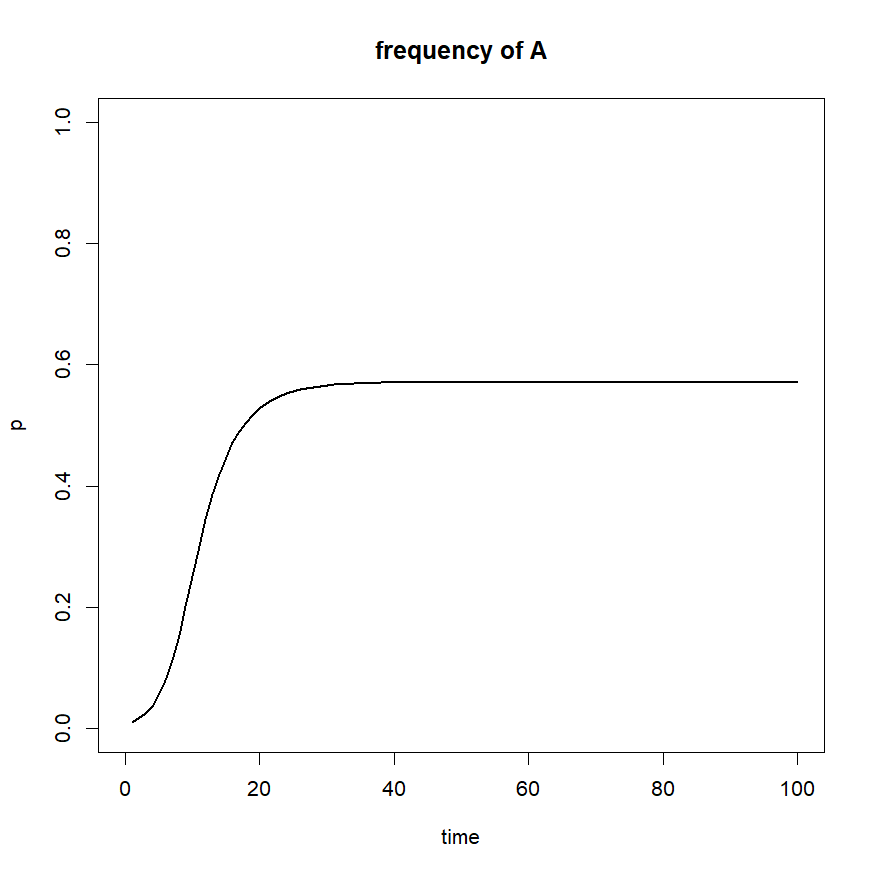``````print(object)
``````
``````##
## Object of class "selection" normally consisting of the expected allele
## frequencies through time under frequency independent selection with genotype
## fitnesses as follows:
##     W(AA) = 0.8
##     W(Aa) = 1.1
##     W(aa) = 0.7
##
## To plot enter plot('object_name') at the command line
## interface.
``````
``````par(mfrow=c(2,2))
plot(object,color="darkgrey",lwd=2)
plot(object,color="darkgrey",show="surface",lwd=2)
plot(object,color="blue",show="deltap")
plot(object,color="darkgrey",show="cobweb")
``````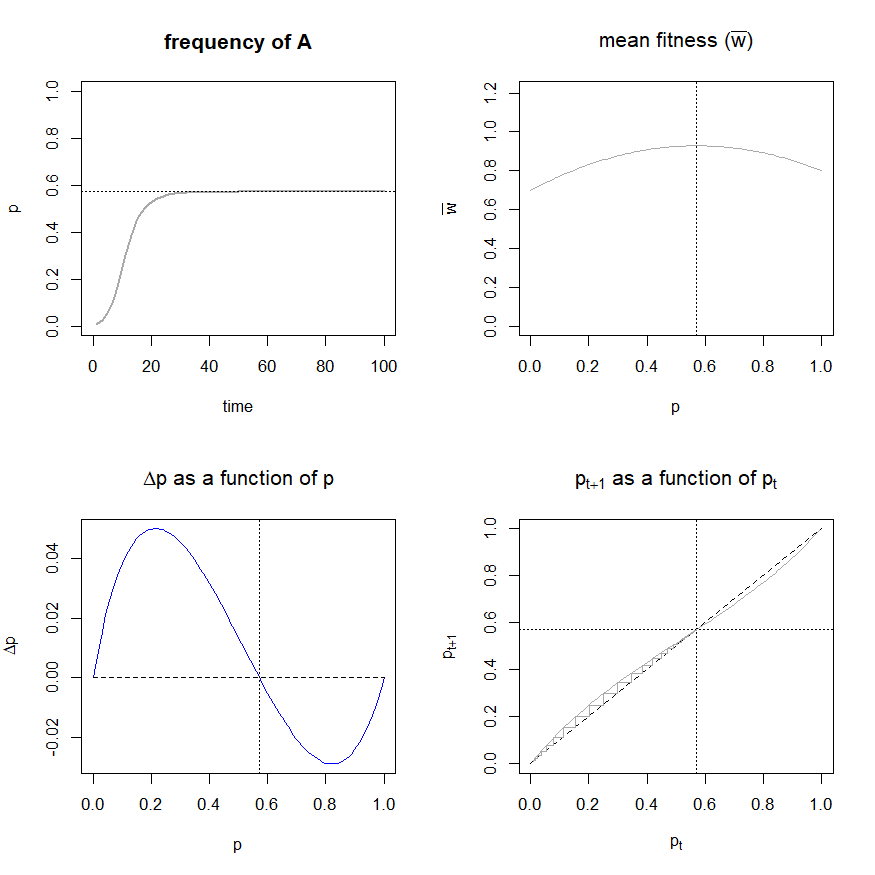## `sexratio`: Hypothetical analysis of frequency dependent selection on a sex determining genetic locus

``````object<-sexratio(p0=0.001,sex.Aa=c(0.9,0.1),
time=20)
``````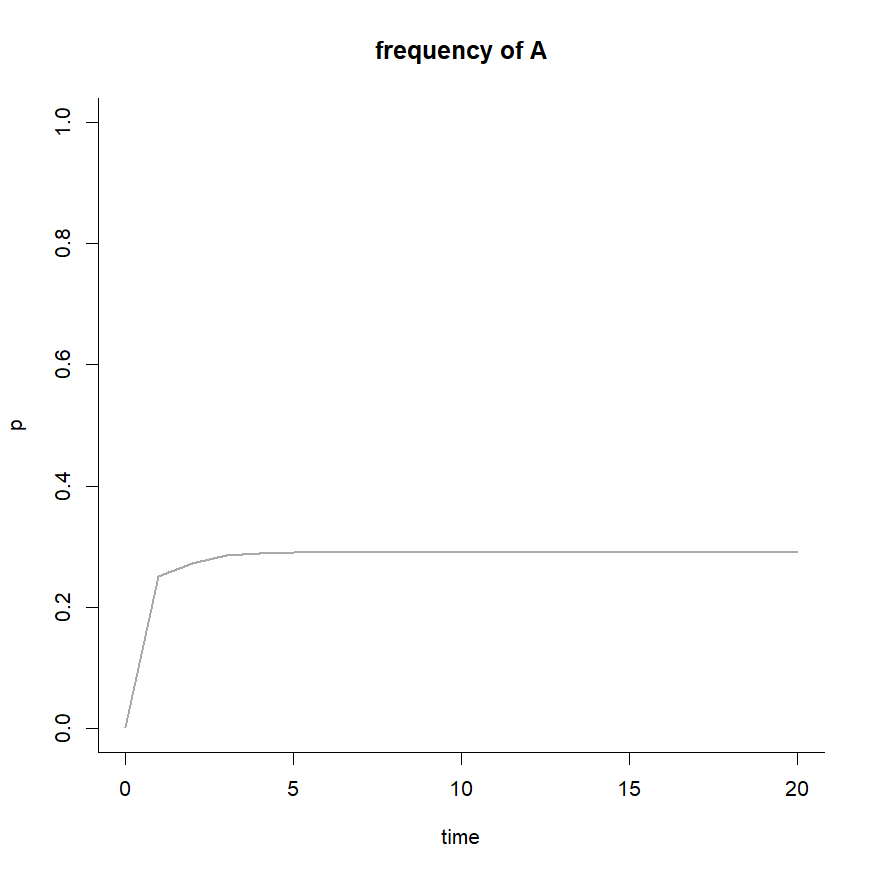``````print(object)
``````
``````##
## Object of class "sexratio" consisting of the expected frequency of the two
## alternative alleles (A & a) at a sex-determination locus in which heterozygotes
## are male with a probability of 0.9; and female with a probability of 0.1.
##
## To plot enter plot('object_name') at the command line
## interface.
``````
``````par(mfrow=c(2,1),mar=c(4.1,4.1,3.1,1.1))
plot(object,lwd=4,type="s",show="sex-ratio")
plot(object,lwd=4,type="s",show="genotypes")
``````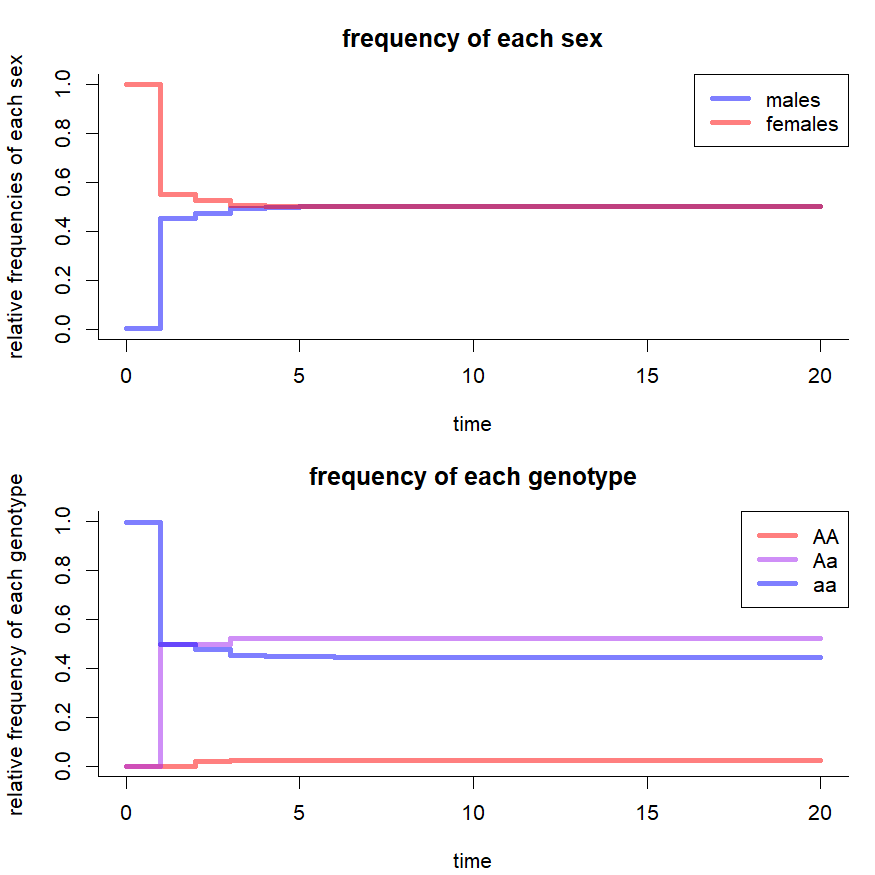``````par(mfrow=c(1,1))
``````

Check back here for more updates, and don't forget that I have also created shiny web application interfaces for various of these functions which can be accessed from their homepage: www.phytools.org/PopGen.

Please let me know if you have or plan to use learnPopGen for teaching. I would love to hear from you!